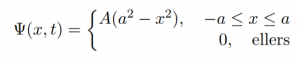# Expectation values of operators for a particle in a given state

A particle has initial wavefunktion:a) Determine the normalization constant $A$.
b) What is the expectation value of $x$ at time $t=0$?
c) What is the expectation value of $p$ at time $t=0$?
d) Find the expectation value of $x^2$.
e) Find the expectation value of $p^2$.
f) Find the uncertainty in $x$ $(\sigma_x)$.